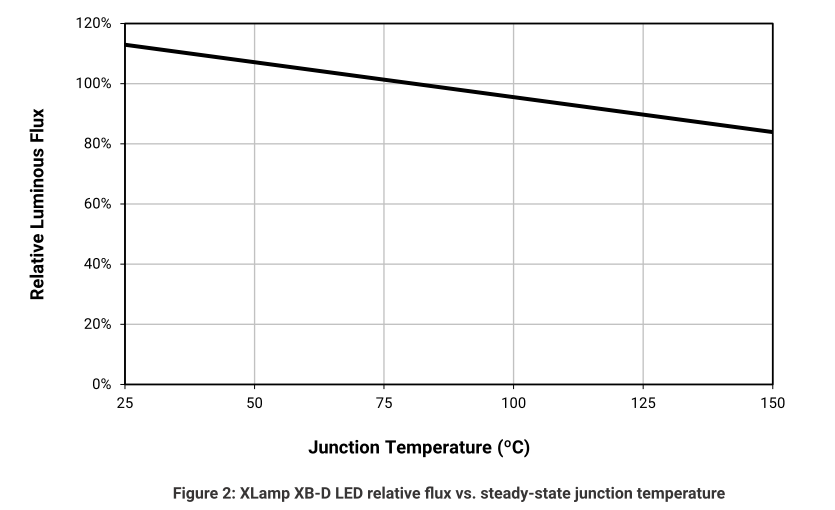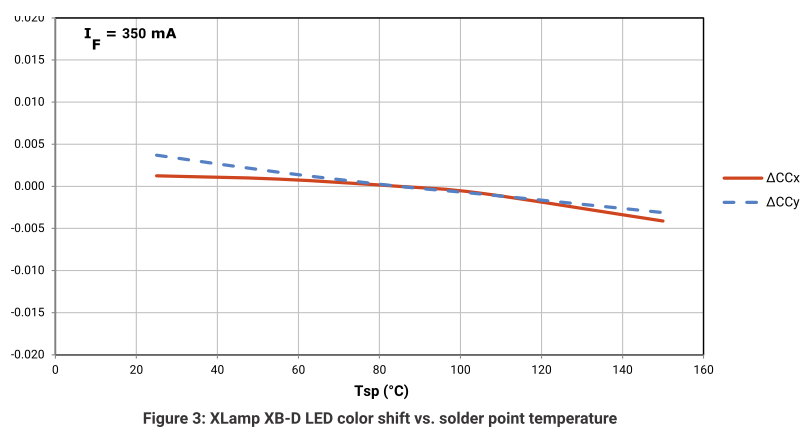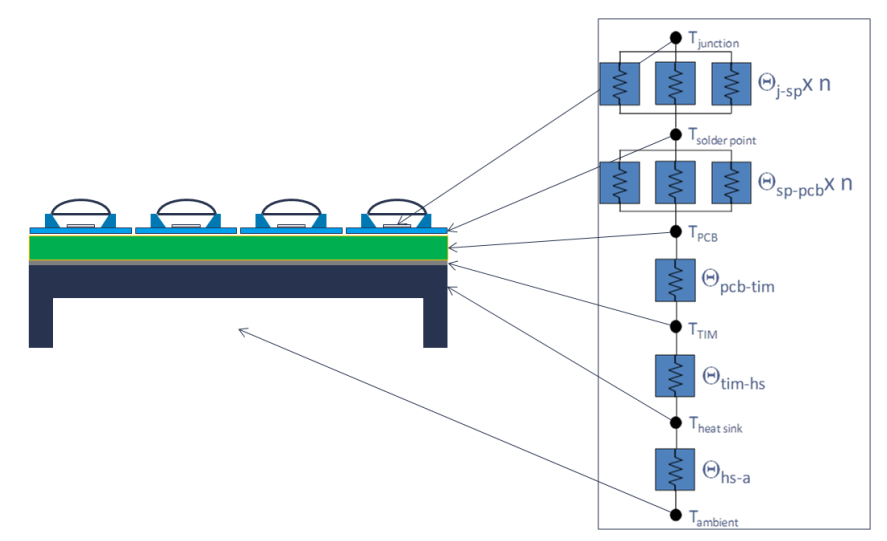Thermal Management for LED COB Grow lights

### Thermal Management for LED COB Grow lights

Renxin.Thermal LED grow lights  lead the industry in brightness and reliability,  enabling  the  LED  lighting  revolution  with  energy-efficient, environmentally friendly light. To take full advantage of the benefits of cob lights, proper thermal manage
Details

Importance of proper theraml management

A main cause of LED failures is improper thermal management. Many performance characteristics of LED components are influenced
by the operating temperature, so LED system designers need a basic understanding of thermal design and performance. All Renxin.Theraml heatsink product datasheets  detail  the  performance  characteristics  that  are  impacted  by  temperature.  Also,  Renxin.Thermal  internally  developed CFD software,  can be used to calculate the specific responses to thermal effects. Some performance characteristics experience a recoverable change, such as RthM ，light output, color and voltage, while others, such as lifetime, can experience a non-recoverable degradation due to high operating temperatures. However, exceeding the maximum operating temperature specification, which is typically a 150 °C junction temperature, can cause permanent and/or catastrophic damage to  LED illuminant , so care must be taken to operate LEDs below this limit.

1、Light output
Elevated junction temperatures cause recoverable light output reduction, which is plotted on each of manufacturer LED datasheets. As
the junction temperature increases, the light output of the LED decreases, but recovers when the LED cools. Below in Figure(1)  is a chart
showing the relative flux versus junction temperature from the XLamp XB-D LED data sheet. The XB-D LED, among many other new
XLamp LEDs, is binned at 85 °C, so the relative flux data is based on 100% light output at an 85 °C junction temperature.2、Color
With increasing junction temperatures, the color of all LEDs shifts. this effect needs to be understood for proper system design. Shown below in Figure（3）again from the XB-D data sheet, are the CCx and CCy shifts relative to solder point temperature (TSP). It is worth noting that the data in this plot shows that there is less than a 0.004 shift in either direction for the full range of operating temperatures.3、 Voltage
Forward voltage decreases as the junction temperature of an LED increases. This is shown on each of  Lamp LED datasheets as
the temperature coefficient of voltage, and varies slightly depending on the color and package type. This value varies from approximately
-1 to -4 mV/°C per LED. It is important to understand the full operating conditions for an LED system so the driver can accommodate the
potential range of drive voltages over the operating temperature of the system. An example of this is shown later in this document.

4、Reliability
The reliability of any LED is a direct function of junction temperature. The higher the  junction  temperature,  the  shorter  the  lifetime  of  the  LED Datasheets  from  an  IES LM-80-08 report can be used to predict the lumen maintenance of an LED under various temperature and drive current operating environments.

Heat Generation

LEDs generate visible light when current passes across the junction of the semiconductor chip. However, LEDs are not 100% efficient,
much of the power running through an LED is output as heat, which thus needs to be dissipated. General blue  LEDs are over 50%
efficient and white  LEDs are over 40% efficient. That is, under normal operating conditions, approximately 50% to 60% of the input
power is output as heat, while the rest of the input power is converted to light. To be conservative, assume LEDs convert 25% of the input
power to light and output 75% of the input power as heat. This estimate varies depending on current density, brightness and component,
but is a good estimate for thermal design. Equation 1 below shows how to calculate the thermal power.

Equation 1: Thermal power calculation  Pt = 0.75  Vf If
Pt  is the thermal power (Watts)
Vf  is the forward voltage of the LED (V)
If    is the source current to the LED (A)
The Vf and If  can be measured directly or calculated from the PCT, so the thermal power can easily be calculated. This is the amount of power the system/heat sink must dissipate.

Heat Transfer
There are three basic modes of heat transfer: conduction, convection and radiation. Each plays a role in LED performance and final  system design and must be understood for proper thermal management

Conduction

Conduction is the transfer of heat through a solid material by direct contact. This is the first mode of heat transfer to get thermal power from the LED junction to the heat sink. Metals are typically the best conductors of heat. The heat conduction potential of all materials can be expressed as thermal conductivity, typically abbreviated as k. Equation 2 below shows how to calculate the quantity of heat transferred  via conduction.

Equation 2: Fourier’s law of heat conduction  Q = K A   (ΔT/ Δx)
is the amount of heat transferred through conduction (W)
K  is the thermal conductivity of the material (W/m K)
A   is the cross sectional area of the material through which the heat flows (m 2 )
ΔT  is the temperature gradient across the material (°C)
Δx  is the distance for the heat must travel (m)

Convection
Convection is the transfer of heat through the movement of fluids and gases. In LED systems, this is typically the transfer of heat from the heatsink to the ambient air. There are two sub-categories of convection: natural and forced. Natural convection occurs with no artificial source of field movement and is due to the buoyancy forces induced by thermal gradients between the fluid and solid. Forced convection occurs when an external instrument such as a fan, pump, or other device is used to artificially move the fluid or gas. In LED cooling systems, convection is the main mode of heat transfer to remove the generated heat from the LED system and heat sink.
Equation 3:  below  shows how to calculate the quantity of heat transferred via convection.

P = H   A  ΔT
P  is the amount of heat transferred through convection (W)
H  is the heat transfer coefficient (W/m 2 K)
A  A is the surface area (m 2 )
ΔT  is  the  temperature  gradient  across  the  material  (°C),  typically  the  difference  between  the  surface  temperature  and ambient air temperature
The fundamental challenge in calculating the heat transfer via conduction is determining and computing the heat transfer coefficient  (h). Typical values for h can vary significantly depending on boundary conditions, geometry and many other factors. However, for natural convection h will usually be in the range of 5-20 W/m 2 K, while for forced convection h can be as high as 100 W/m 2 K for air and up to 10,000 W/m 2 K for water. Typically, for natural convection in air, a value of 10 W/m 2 K is a good assumption for an initial rough calculation.

The transfer of thermal energy through an electromagnetic field is the third component of heat transfer, radiation. The magnitude of  radiation heat transfer is based on the emissivity of the material, which is the ratio of how closely the surface approximates a blackbody. In an LED system, radiation typically has a very small effect on the net system heat transfer since the surface areas are typically fairly  small and surface temperatures are relatively low, to keep the LED junction temperatures below the maximum rated temperature of 150 °C. Equation 4 below shows how to calculate the quantity of heat transferred via radiation

Equation 4  Radiative heat transfer equation  Q =  ε  σ  A  (Ts4  - Tf4 )

Q  is the amount of heat transferred through radiation (W)
ε is the emissivity of the surface (dimensionless)
σ is the Stefan-Boltzmann constant (5.67 x 10 -8  W/m 2 K 4 )
A is the surface area (m 2 )
Ts  is the surface temperature of the material (°C)
Tf is the fluid temperature of the medium (°C), typically referenced to the ambient air temperature

Thermal Model
The thermal model of an LED system can be illustrated by a simple resistor network similar to an electrical circuit. Thermal resistances
are represented by the resistors, the heat flow is approximated by the electrical current, and the corresponding temperatures within the
system correspond to the electrical voltages. Below in Figure (4) is a resistor network representation of a multiple-LED system on a printed
circuit board (PCB) mounted to a heat sink in ambient air.is the temperature at each corresponding location (°C)
Θa-b  is the thermal resistance from point a to point b (°C/W)
is the number of LED components on a single PCB
To summarize the heat path illustrated in the Figure (4) above, heat is conducted from the LED junctions through the LED components to the PCB, through the thermal interface material (TIM) to the heat sink and then convected and radiated to the ambient air.The nodes in the circuit represent the individual sections within the system and the locations where temperatures may be measured. For example, the solder point temperature  (Tsolder point)  represents the location on the board, as specified in the corresponding data sheet for each Cree XLamp LED, where the temperature on the top of the PCB can be measured. This can be used to calculate the junction temperature, which is detailed in a later section.The resistors represent the thermal resistances of the individual contributors. For example, ΘJ-SP represents the thermal resistance of the LED component from junction to solder point.The system is divided into a network of parallel connections for the multiple LEDs and series connections for the singular components. If the system includes only one LED, or n =1 in Figure (3), the entire thermal path is simply in series.